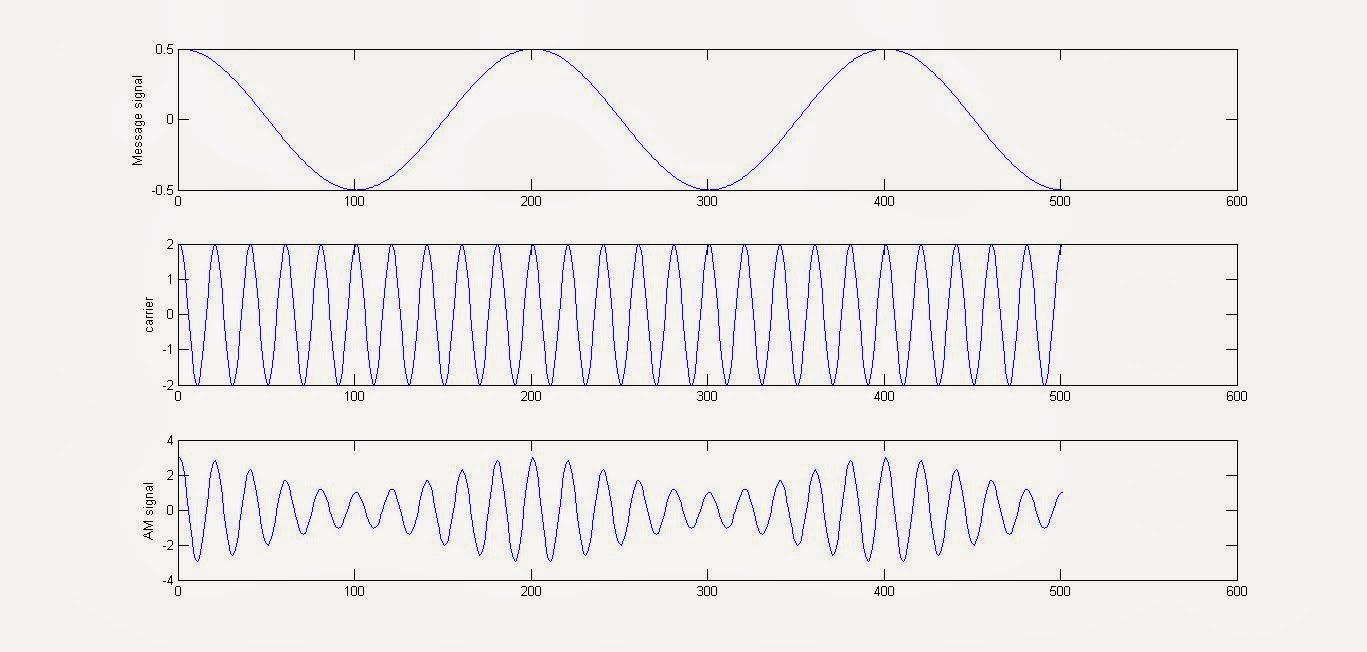# MATLAB Code to Simulate / Generate the Amplitide Modulation Signal (AM Signal) [ Amplitude Modulation using MATLAB]

Amplitude modulation (AM) is a method of superimposing the message onto an alternating carrier waveform.The highest frequency of the modulating signal (Message Signal) is normally < 10 % of the carrier frequency. The instantaneous amplitude of the modulated wave varies as per the amplitude of the message signal.

MATLAB Code For Amplitude Modulation:

clc;
clear all;
close all;

Ac=2;
%carrier amplitude
fc=0.5; %carrier frequency
Am=.5; %message signal amplitude
fm=.05; %message signal frequency
Fs=100; %sampling rate/frequency

ka=1;
%Amplitude Sensitivity

t=[0:0.1:50];
%defining the time range & disseminating it into samples
ct=Ac*cos(2*pi*fc*t); %defining the carrier signal wave
mt=Am*cos(2*pi*fm*t); %defining the message signal
AM=ct.*(1+ka*mt); %Amplitude Modulated wave, according to the standard definition

subplot(3,1,1);
%plotting the message signal wave
plot(mt);
ylabel('Message signal');

subplot(3,1,2);
%plotting the carrier signal wave
plot(ct);
ylabel('carrier');

subplot(3,1,3);
%plotting the amplitude modulated wave
plot(AM);
ylabel('AM signal');

Screenshot of the output:MATLAB Code to Simulate / Generate the Amplitide Modulation Signal (AM Signal) [ Amplitude Modulation using MATLAB]

Visit More On Digital iVision Labs:
#FSK Simulation Code Using MATLAB
#Phase Shift Keying (PSK) Modulation MATLAB Simulation, With MATLAB Code

1.How can we interface this code with arduino uno kit

1.Use Serial Communication for sending the digits to Arduino And use analog pin to create a corresponding voltage: Idea for that: http://www.divilabs.com/2013/11/accessing-serial-port-using-matlab-code.html
Although MATLAB has also developed a Arduino Toolkit that can help you in this regard.

2.Hi,
what is modulation coefficient? Did you mean modulation Index by it? What is the use of 'Fs' in the code?
Regards
Nazmul

1.ka is Amplitude Sensitivity. I have corrected it. Fs is sampling rate. For setting a sampling rate we need to follow the Niquist Criteria. Which needs it to be atleast twice the maximum frequency component if the signal.

2.ka should be a positive value such that, Ac.*(1+ka*mt) is greater than or zero (i.e., non negative).

3.Hi,
What is modulation coefficient? Did you mean Modulation Index by it? What is the use of 'Fs' in the code?

1.ka is Amplitude Sensitivity. I have corrected it. Fs is sampling rate. For setting a sampling rate we need to follow the Niquist Criteria. Which needs it to be atleast twice the maximum frequency component if the signal.

4.